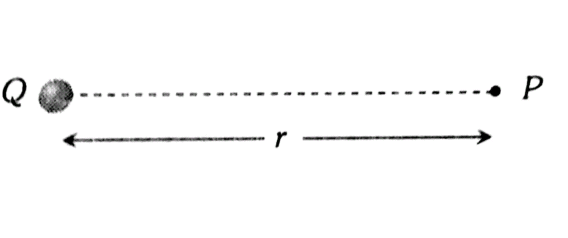#### If we merged 125 spherical mercury droplets having 2.5 V potential of each mercury droplet. What will be the potential of entire droplets will be? Option 1) 0.1 V Option 2) 1.0 V Option 3) 62.5 V Option 4) 4 V

As we learnt in

Electric field and potential Due to various charge Distribution i) Point Charge -

Electric field and Potential at Point P due to a Point charge Q.,- whereinLet radius of each small drop is r and radius of bigger drop be R.Initial charge = q

Final Charge = 125 qOption 1)

0.1 V

This option is incorrect

Option 2)

1.0 V

This option is incorrect

Option 3)

62.5 V

This option is correct

Option 4)

4 V

This option is incorrect

#### Vakul

v' = nv

given, n= 125

therefore, 125= 25

that is, v' = 25 * 2.5 = 62.5